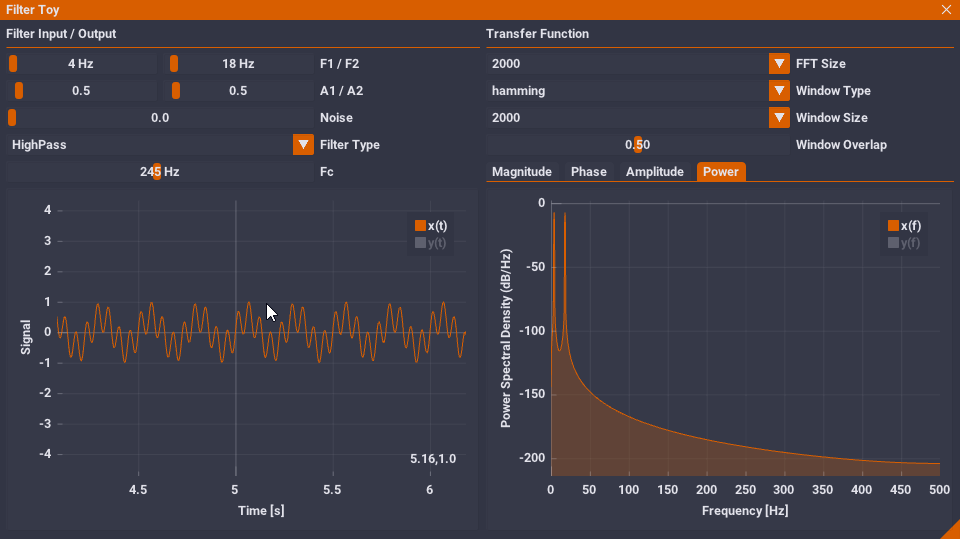etfe

# etfe

`ETFE.hpp` emulates MATLAB's `tfestimate`, `pwelch`, and `cpsd` functions. It calculates the experimental transfer function estimate between input x and output y `txy`, the power spectral densities `pxx` and `pyy`, and the cross spectral density `pxy`. By default, it behaves exactly as MATLAB's functions, and similarly can be provided with specified windows, overlap, and FFT sizes. The output has been tested to precisely match that of MATLAB's (see `matlab\test.m`).

Note: Currently, only real-valued 1D sample inputs are supported.

## Usage

MATLAB

```[pxx,f] = pwelch(x,[],[],[],fs);
[pxy,f] = cpsd(x,y,[],[],[],fs);
[txy,f] = tfestimate(x,y,[],[],[],fs);
mag     = 20*log10(abs(txy));
phase   = 180/pi*angle(txy);```

ETFE.hpp

```ETFE etfe(nsamples,fs);
ETFE::Result& result = etfe.estimate(x,y);

result.f;
result.txy;
result.pxx;
result.pxy;
result.mag;
result.phase;```

## Example Apps

• `etfe_cl.cpp` - command line interface to compute ETFE from CSV files
• `filter_toy.cpp` - interactive spectrum and Bode plots for filters## Dependencies

• KISS FFT - other FFT packages should be easily substitutable
• mahi-gui - for filter demo only
• IIR - for filter demo only

## Resources

• #### crush

when setup passed (5x48000,48000,hanning(2^14),2^14x0.75,2^14). like [sensor_spectrum, freq] = pwelch(signal,w,NOVERLAP,NFFT,Fs); in matlab

opened by SunnyTian 0
###### Evan Pezent
PhD candidate studying robotics in the Mechatronics and Haptic Interfaces Lab at Rice University.###### The Universal Storage Engine

The Universal Storage Engine TileDB is a powerful engine for storing and accessing dense and sparse multi-dimensional arrays, which can help you model

1.1k Jun 14, 2021
###### http://torch.ch

Development Status Torch is not in active developement. The functionality provided by the C backend of Torch, which are the TH, THNN, THC, THCUNN libr

8.6k Jun 11, 2021
###### Primary repository for the Trilinos Project

Trilinos Website | Documentation | Mailing List | Packages The Trilinos Project is an effort to develop algorithms and enabling technologies within an

640 Jun 14, 2021
###### C++ implementation of the Python Numpy library

NumCpp: A Templatized Header Only C++ Implementation of the Python NumPy Library

1.7k Jun 12, 2021
###### C++ library for solving large sparse linear systems with algebraic multigrid method

AMGCL AMGCL is a header-only C++ library for solving large sparse linear systems with algebraic multigrid (AMG) method. AMG is one of the most effecti

419 Jun 10, 2021